# Right triangle

Right triangle ABC with side a = 19 and the area S = 95. Calculate the length of the remaining sides.

b =  10
c =  21.5

### Step-by-step explanation:

$b=\frac{2S}{a}=\frac{2\cdot 95}{19}=10$
$c=\sqrt{1{9}^{2}+{b}^{2}}=21.5$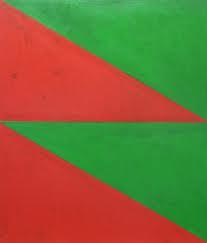Did you find an error or inaccuracy? Feel free to write us. Thank you!Tips to related online calculators

#### You need to know the following knowledge to solve this word math problem:

We encourage you to watch this tutorial video on this math problem:

## Related math problems and questions:

• Squares above sidesTwo squares are constructed on two sides of the ABC triangle. The square area above the BC side is 25 cm2. The height vc to the side AB is 3 cm long. The heel P of height vc divides the AB side in a 2: 1 ratio. The AC side is longer than the BC side. Calc
• Triangle ABCIn a triangle ABC with the side BC of length 2 cm The middle point of AB. Points L and M split the AC side into three equal lines. KLM is an isosceles triangle with a right angle at the point K. Determine the lengths of the sides AB, AC triangle ABC.
• ParallelogramCalculate area of the parallelogram ABCD as shown if |AB| = 19 cm, |BC| = 18 cm and angle BAD = 90°
• Triangle ABCCalculate the sides of triangle ABC with area 1404 cm2 and if a: b: c = 12:7:18
• SAS triangleThe triangle has two sides long 7 and 19 and included angle 36°. Calculate area of this triangle.
• The farmerThe farmer would like to first seed his small field. The required amount depends on the seed area. Field has a triangular shape. The farmer had fenced field, so he knows the lengths of the sides: 119, 111 and 90 meters. Find a suitable way to determine th
• TriangleCalculate the area of ​​the triangle ABC if b = c = 17 cm, R = 19 cm (R is the circumradius).
• Equilateral triangleCalculate the side of an equilateral triangle if its area is 892 mm2.
• Rectangle - sides 3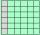If in the rectangle ABCD we enlarge the side a by 5 cm and decrease the side b by 2 cm, the rectangle area will be reduced by 5 cm². When we decrease the length of the side a by 4 cm and at the same time we increase the length of side b by 3 cm we increas
• TriangleCalculate heights of the triangle ABC if sides of the triangle are a=82, b=44, and c=53.
• Circle in rhombus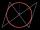In the rhombus is an inscribed circle. Contact points of touch divide the sides to parts of length 19 cm and 6 cm. Calculate the circle area.
• Triangle - is RT?Triangle has a circumference of 90 cm. Side b is 1 cm longer than c, and side c is 31 cm longer than side a. Calculate the length of sides and determine whether a triangle is a right triangle.
• Triangle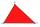For how many integer values of x can 6, 19 and x be the lengths of the sides of triangle?
• Triangle KLBIt is given equilateral triangle ABC. From point L which is the midpoint of the side BC of the triangle it is drwn perpendicular to the side AB. Intersection of perpendicular and the side AB is point K. How many % of the area of the triangle ABC is area o
• The farmer field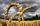The field has a parallelogram shape with dimensions side a = 80 m and height 296 m. Two and two sides is at angle 80°. Calculate acreage of field in hectares.
• The triangles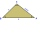The triangles KLM and ABC are given, which are similar to each other. Calculate the lengths of the remaining sides of the triangle KLM, if the lengths of the sides are a = 7 b = 5.6 c = 4.9 k = 5
• Sss triangleCalculate the area and heights in the triangle ABC by sides a = 8cm, b = 11cm, c = 12cm